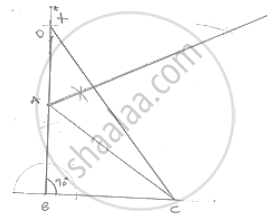# Construct a Right Triangle Abc Whose Base Bc is 6 Cm and the Sum of Hypotenuse Ac and Other Side Ab is 10 Cm. - Mathematics

Construct a right triangle ABC whose base BC is 6 cm and the sum of hypotenuse AC and other side AB is 10 cm.

#### SolutionSteps of construction:
Step 1: Draw a line segment BC of 6cm.
Step 2: At B draw an angle le × BC of 90° .
Step 3: with center B and radius 10cm draw an arc which intersects XB at D.
Step 4: Join DC.
Step 5: Draw the perpendicular bisector of DC which intersects DB at A
Step 6: Join AC
∴ΔABC is the required triangle

Concept: Some Constructions of Triangles
Is there an error in this question or solution?

#### APPEARS IN

RD Sharma Mathematics for Class 9
Chapter 16 Constructions
Exercise 16.3 | Q 7 | Page 18

Share